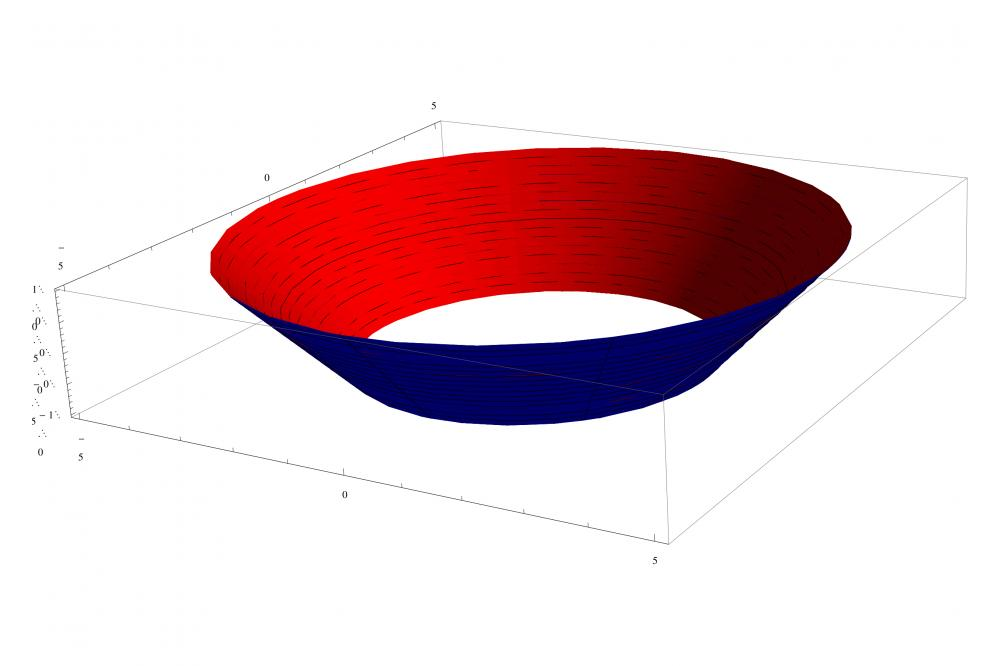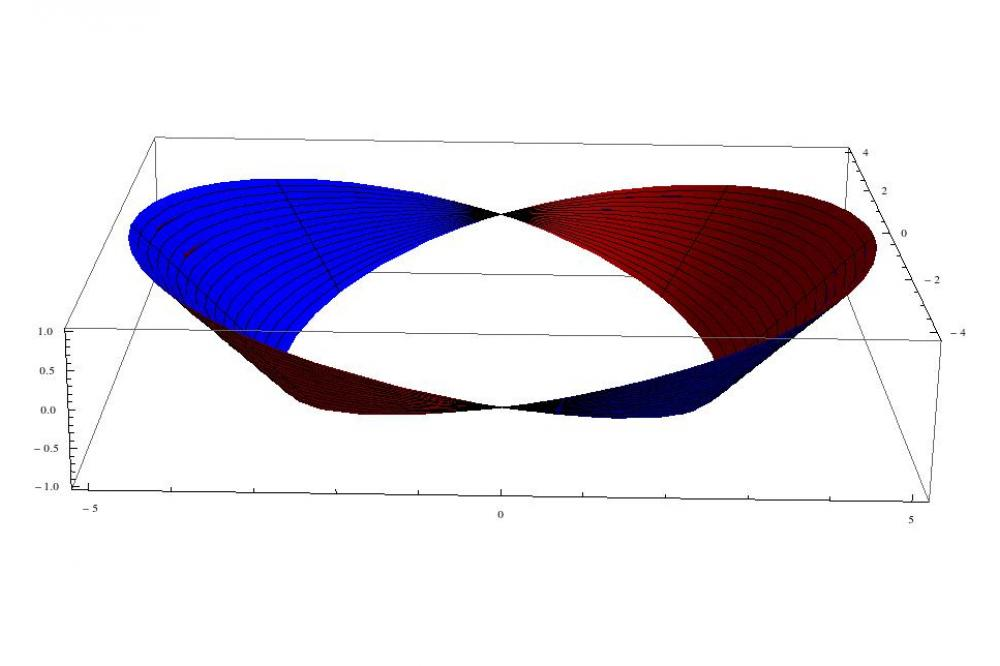# Extracting Hadron Masses from Lattice QCD Simulations at Fixed TopologyExtracting Hadron Masses 1

Fig. 1: Closed strip with trivial topology (Q=0).Extracting Hadron Masses 2

Fig. 2: Closed strip with nontrivial topology (Q=+1).

## Introduction

Topology is an important concept in physics, e.g. in field theory, in condensed matter physics or in cosmology. Topology characterizes the shape of an object in terms properties that are preserved under continuous deformations including stretching and bending, but not tearing or gluing.

Two simple strips of paper can serve as an illustrative example. Take the first one and glue its two ends together without introducing any unneccessary turns (like closing a belt; cf. Fig. 1). Proceed in a similar way with the second strip, but make a full turn with one end, before attaching it to the other end (cf. Fig. 2). The two strips have now a different topological structure. It is not possible to deform the second strip to look exactly like the first strip without cutting the strip and removing the winding.

This idea can be generalized in a straightforward way. To each closed strip one can associate a winding number or topological charge Q, which counts the number of left handed turns minus the number of right handed turns (Fig. 1 corresponds to Q=0, Fig. 2 to Q=+1). Two strips with the same topological charge can be deformed continuously into each other. If two strips have different topological charges, this is not possible.

## Methods

Quantum chromoynamics (QCD) is the theory of quarks and gluons, which for example explains the formation and the mass of protons and neutrons. The gluons, which bind the quarks together, are described by a field, which is similar to the well-known electromagnetic field. Similar to the peviously discussed closed strip of paper also gluonic field configurations can exhibit windings, instantons, and anti-instantons. These (anti-) instantons cannot be removed or generated by continuous deformations of the gluonic field configuration. They are localized in space and in time and hence can be interpreted as particles formed by gluons with a very short life time (they are also called pseudoparticles). The number of instantons minus the number of antiinstantons is the topological charge of a gluonic field configuration. Lattice QCD is a method to solve the extremely complicated equations of QCD numerically.

To be able to compute a physical quantity, for example the proton mass, one needs to generate a representative set of gluonic field configurations with many different topological charges with Monte Carlo algorithms.

## Results

We are among the first to test these methods in practice, by considering models, which are simpler, but similar to QCD: quantum mechanics on a circle, the 2D Schwinger model and SU(2) Yang-Mills theory. Recently, we have also improved the methods from a theoretical point of view, by devolping higher order (i.e. preciser) equations.[1-4]

## Discussion

Unfortunately, these algorithms have severe difficulties in changing the topological charge of a gluonic field configuration, in particular, when the lattice spacing is small. Due to this problem, which is called topology freezing, it is of interest, to develop methods, to compute physical quantities like the proton mass from a set of gluonic field configurations from a single topological sector. Such methods have been suggested some time ago.

## Last Update

• Last Update: 2020-03-05 14:40